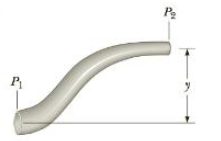Chapter 9, Problem 42P

Chapter
Section
Textbook Problem

Water moves through a constricted pipe in steady, ideal flow. At the lower point shown in Figure P9.42, the pressure is 1.75 × 105 Pa and the pipe radius is 3.00 an. At the higher point located at y =2.50 m, the pressure is 1.20 × 105 Pa and the pipe radius is 1.50 cm. Find the speed of flow (a) in the lower section and (b) in the upper section, (c) Kind the volume flow rate through the pipe.Figure P9.42

(a)

To determine
The speed of water flow in the lower section.

Explanation
Bernoulli’s equation is used for the speed of water flow in the lower section as P1+(1/2)ρwv12+ρwgy2=P2+(1/2)ρwv22+ρwgy2v22v12=2[P1P2ρwg(y2y1)] but the speed of the water flow in upper section is obtained from the equation of continuity as v2=(A1/A2)v1=(r1/r2)2v1 . Now, the flow speed of water in the lower is [(r1/r2)41]v12=2[P1P2ρwg(y2y1)]v1=2[P1P2ρwg(y2y1)]/[(r1/r2)41] .

Given info: The pressure at the lower point is 1.75×105Pa , the pressure at higher point is 1.20×105Pa , density of water is 1.00×103kg/m3 , the height difference is 2.50m , acceleration due to gravity is 9.80m/s2 , radius of lower section is 3.00cm , and radius of higher section is 1.50cm

(b)

To determine
The speed of water flow in the upper section.

(c)

To determine
The volume flow rate through the pipe.

Still sussing out bartleby?

Check out a sample textbook solution.

See a sample solution

The Solution to Your Study Problems

Bartleby provides explanations to thousands of textbook problems written by our experts, many with advanced degrees!

Get Started

Find more solutions based on key concepts